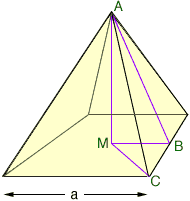Subject: pyramids I'm in tenth grade and I know that there's some formula for finding out the slope of the side of a pyramid. I thought it could be the pythagorean therom, but I'm not sure if this works. Please send me the formula as soon as possible, I need it for a project. Kelly Hi Kelly, In the diagram below |AM| is the height of the pyramid.If by "the slope of the side of a pyramid" you mean the slope of the line AB then it is the height, |AM|, divided by a/2. If you want the slope of the line AC then this is |AM| divided by |MC|. If you can measure the height |AM| and the side length |AC| then you can use the right triangle AMC and the pythagorean theorem to find |MC| form |AC|2 = |AM|2 + |MC|2 Triangles AMB and CBM are also right triangles so you might use the pythagorean theorem with them. Which triangle you use depends on what you know or what you can measure. I hope this helps, Harley Go to Math Central